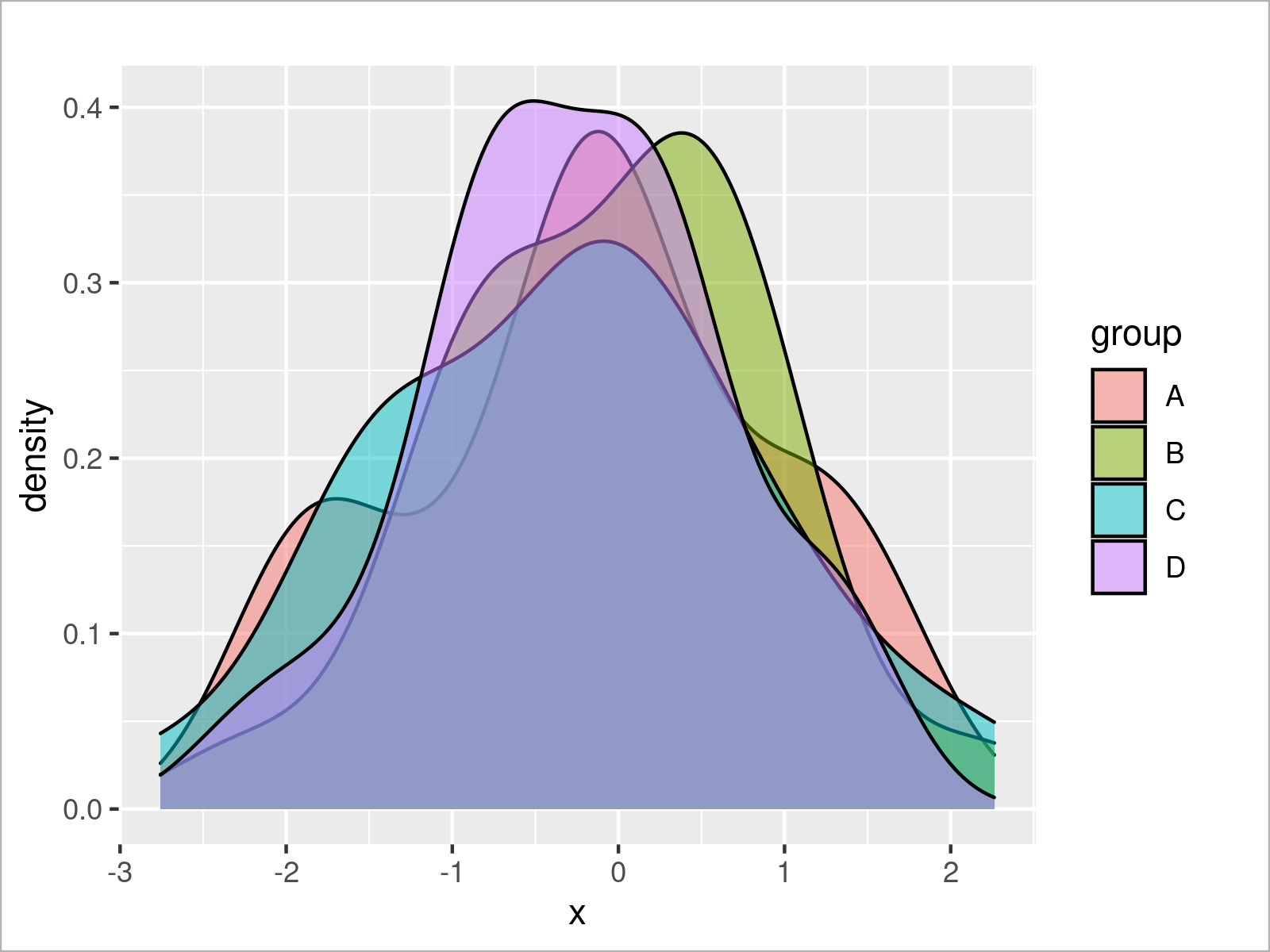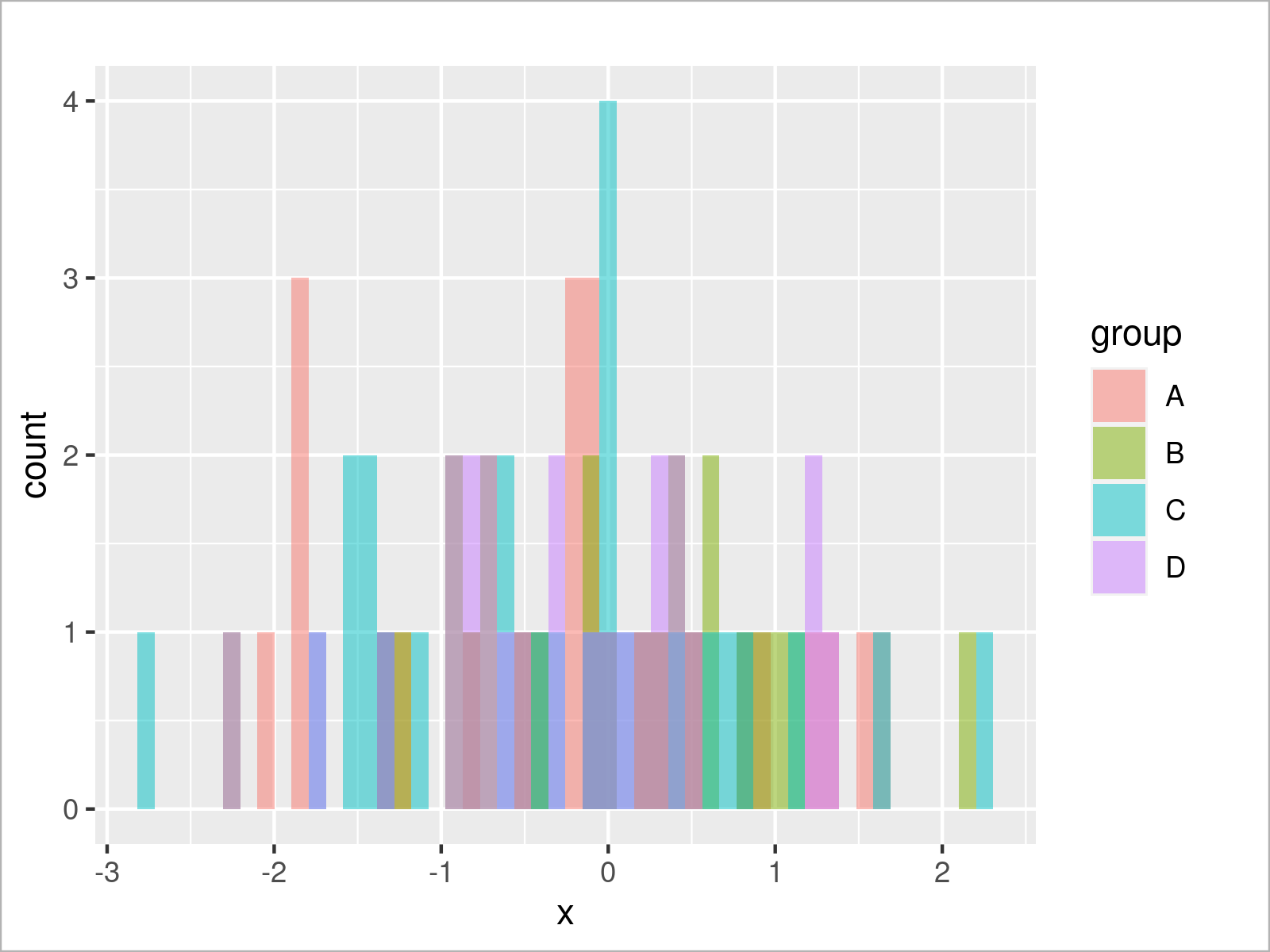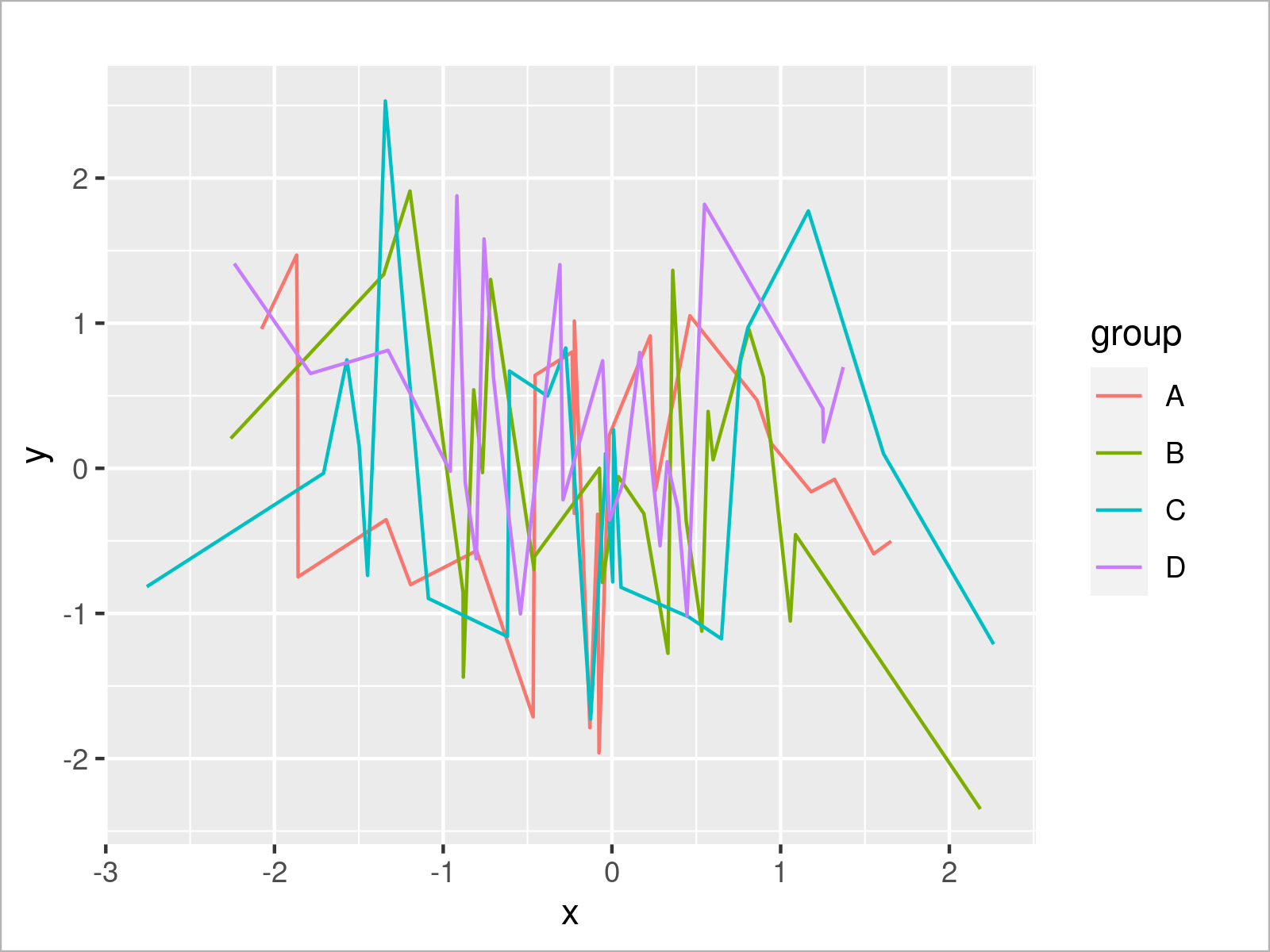# theme_gray ggplot2 Theme in R (6 Examples)

In this R tutorial you’ll learn how to change the ggplot2 theme to the theme_gray.

The content of the page is structured like this:

You’re here for the answer, so let’s get straight to the examples:

## Example Data & Add-On Packages

We use the following data as basement for this R tutorial:

```set.seed(842236)                           # Set random seed
data <- data.frame(x = rnorm(100),         # Create example data frame
y = rnorm(100),
group = LETTERS[1:4])Have a look at the table that got returned after running the previous R code. It reveals the first six rows of the example data, and that our data is constituted of three columns. The columns x and y are numerical and the variable group has the character data type.

In case we want to use the functions of the ggplot2 package, we also have to install and load ggplot2:

```install.packages("ggplot2")                # Install ggplot2 package

In the following examples, we’ll draw different kinds of graphics using the theme_gray (also called theme_grey) of the ggplot2 package.

Note that this is the default theme of the ggplot2 package. For that reason, we could simply remove the theme_gray code from the examples below to produce the same output graphics.

Anyway, let’s draw some plots!

## Example 1: Draw ggplot2 Scatterplot Using theme_gray()

```ggplot(data,                               # theme_gray scatterplot
aes(x = x,
y = y,
col = group)) +
geom_point() +
theme_gray()```## Example 2: Draw ggplot2 Density Plot Using theme_gray()

```ggplot(data,                               # theme_gray density plot
aes(x = x,
fill = group)) +
geom_density(alpha = 0.5) +
theme_gray()```## Example 3: Draw ggplot2 Histogram Using theme_gray()

```ggplot(data,                               # theme_gray histogram
aes(x = x,
fill = group)) +
geom_histogram(alpha = 0.5,
position = "identity",
bins = 50) +
theme_gray()```## Example 4: Draw ggplot2 Barchart Using theme_gray()

```ggplot(aggregate(y ~ group, data, sum),    # theme_gray barplot
aes(x = group,
y = y,
fill = group)) +
geom_bar(stat = "identity") +
theme_gray()```## Example 5: Draw ggplot2 Boxplot Using theme_gray()

```ggplot(data,                               # theme_gray boxplot
aes(x = x,
fill = group)) +
geom_boxplot() +
theme_gray()```## Example 6: Draw ggplot2 Line Plot Using theme_gray()

```ggplot(data,                               # theme_gray line plot
aes(x = x,
y = y,
col = group)) +
geom_line() +
theme_gray()```## Video & Further Resources

Would you like to learn more about the ggplot2 package and its variety of functions, then please check out the video below, where you get a detailed introduction to the package and data visualization in R.

I have also created tutorials on how to use other ggplot2 themes in R:

Furthermore, you may have a look at the introductions to other add-on packages that provide extra themes for ggplot2 plots:

Also, you could have a look at the other tutorials on my website. Some tutorials are shown below.